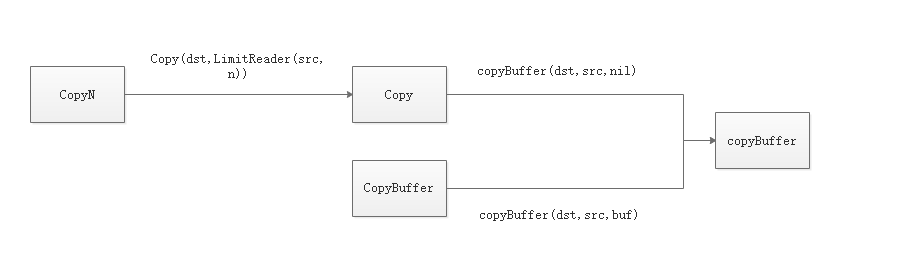# io包 copy - golang

aixinaxc · · 2864 次点击 · · 开始浏览

在io包中主要是操作流的一些方法，今天主要学习一下copy。

在io包（golang 版本 1.12）中，提供了3个公开的copy方法：CopyN(),Copy(),CopyBuffer().

CopyN(dst,src,n) 为复制src 中 n 个字节到 dst。

Copy（dst,src） 为复制src 全部到 dst 中。

CopyBuffer（dst,src,buf）为指定一个buf缓存区，以这个大小完全复制。

他们的关系如下：从图可以看出，无论是哪个copy方法最终都是由copyBuffer（）这个私有方法实现的。下面我们看看这个方法的源码。

``````func copyBuffer(dst Writer, src Reader, buf []byte) (written int64, err error) {
// If the reader has a WriteTo method, use it to do the copy.
// Avoids an allocation and a copy.
if wt, ok := src.(WriterTo); ok {
return wt.WriteTo(dst)
}
// Similarly, if the writer has a ReadFrom method, use it to do the copy.
if rt, ok := dst.(ReaderFrom); ok {
}
if buf == nil {
size := 32 * 1024
if l, ok := src.(*LimitedReader); ok && int64(size) > l.N {
if l.N < 1 {
size = 1
} else {
size = int(l.N)
}
}
buf = make([]byte, size)
}
for {
if nr > 0 {
nw, ew := dst.Write(buf[0:nr])
if nw > 0 {
written += int64(nw)
}
if ew != nil {
err = ew
break
}
if nr != nw {
err = ErrShortWrite
break
}
}
if er != nil {
if er != EOF {
err = er
}
break
}
}
return written, err
}``````

从这部分代码可以看出，复制主要分为3种。

``````if buf == nil {
size := 32 * 1024
if l, ok := src.(*LimitedReader); ok && int64(size) > l.N {
if l.N < 1 {
size = 1
} else {
size = int(l.N)
}
}
buf = make([]byte, size)
}``````0 回复

• 请尽量让自己的回复能够对别人有帮助
• 支持 Markdown 格式, **粗体**、~~删除线~~、``单行代码``
• 支持 @ 本站用户；支持表情（输入 : 提示），见 Emoji cheat sheet
• 图片支持拖拽、截图粘贴等方式上传

在io包中主要是操作流的一些方法，今天主要学习一下copy。

在io包（golang 版本 1.12）中，提供了3个公开的copy方法：CopyN(),Copy(),CopyBuffer().

CopyN(dst,src,n) 为复制src 中 n 个字节到 dst。

Copy（dst,src） 为复制src 全部到 dst 中。

CopyBuffer（dst,src,buf）为指定一个buf缓存区，以这个大小完全复制。

他们的关系如下：从图可以看出，无论是哪个copy方法最终都是由copyBuffer（）这个私有方法实现的。下面我们看看这个方法的源码。

``````func copyBuffer(dst Writer, src Reader, buf []byte) (written int64, err error) {
// If the reader has a WriteTo method, use it to do the copy.
// Avoids an allocation and a copy.
if wt, ok := src.(WriterTo); ok {
return wt.WriteTo(dst)
}
// Similarly, if the writer has a ReadFrom method, use it to do the copy.
if rt, ok := dst.(ReaderFrom); ok {
}
if buf == nil {
size := 32 * 1024
if l, ok := src.(*LimitedReader); ok && int64(size) > l.N {
if l.N < 1 {
size = 1
} else {
size = int(l.N)
}
}
buf = make([]byte, size)
}
for {
if nr > 0 {
nw, ew := dst.Write(buf[0:nr])
if nw > 0 {
written += int64(nw)
}
if ew != nil {
err = ew
break
}
if nr != nw {
err = ErrShortWrite
break
}
}
if er != nil {
if er != EOF {
err = er
}
break
}
}
return written, err
}``````

从这部分代码可以看出，复制主要分为3种。

``````if buf == nil {
size := 32 * 1024
if l, ok := src.(*LimitedReader); ok && int64(size) > l.N {
if l.N < 1 {
size = 1
} else {
size = int(l.N)
}
}
buf = make([]byte, size)
}``````